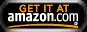Reviews of books by Benoit Mandelbrot BENOIT B. MANDELBROT (1924-2010) developed the theory of fractal geometry. He worked for IBM and various research institution and is a professor emeritus from Yale University. The Fractal Geometry of Natureby Benoit B. Mandelbrot   W. H. Freeman; 1 edition (1983) This essay written in 1975, extended in '77 and published in its current form in 1983 is Mandelbrot's personal account of how he came to develop the idea of fractal dimensions, i.e., the treatment of 'mathematical monsters' that describe seemingly finite geometric forms (such as the length of a coast line or national border), but are in fact indeterminately long, if one decreases the size of the measurement unit used to determine its dimension. Interestingly, all forms in nature appear to be fractal rather than Euclidean, meaning they can be approximated by continuous and finite geometric forms such as lines, circles and spheres (and their related topologies), but are in reality very different. Outlines and surfaces of natural objects are best described by a fractal dimension (2.35 dimensional), rather than an integer dimension (e.g. two dimensional, three dimensional). The most intriguing fact about this mathematical treatment of natural forms is the realization that fractal geometry mimics rugged and chaotic forms like river beds, mountain ranges, tree branches, blood vessels, molecular motion etc.) that are hard to model by conventional (Euclidean) geometry, i.e., functions with derivatives. Fractal dimensions do not have derivatives, because the underlying geometry changes with a changing scale. While fractals do not have derivatives, they can be created by very simple functions similar to Wolfram's cellular automatons (see A New Kind of Science). The emphasis here is on making the structures rather than deriving them by approximation from functions with derivatives. Mandelbrot indeed urges us to see Nature and its forms in a new light. He asks us to see not how something looks, but what it takes to make it (indeed all forms of life originate from a single cell). Thus, natural things form by simple rules (again fractal equations or cellular automatons). Understanding these rules used to grow organisms is the new challenge in our pursuit of understanding of what life is. A fascinating account of Mandelbrot's discovery and development of fractals is shown in the 2008 PBS NOVA video 'Fractals - hunting the hidden dimensions. Watch it online at PBS NOVA. June 24, 2010 /  © 2010 Lukas K. Buehler / go back to Book Review Home